amortization.com Ltd.
Burlington, Ontario

905-639-0374
905-407-7988

info@amortization.comBorrower Beware

Monthly payment mortgages using the 365 day year, exact day monthly interest factor method, have a slightly higher effective interest rate (EIR).

Always ask for an amortization schedule when you borrow money. To be absolutely in the know, also ask for the annual interest rate to be quoted to 4 decimal places.

Bob and Mary, a young married couple purchase their first home and obtain a 30 year \$100,000 mortgage and neglect to ask for an amortization schedule before signing the deal. Below is one of the possible amortization schedules they could have been given.

(Screenshot 1)

The spreadsheet schedule shown is based upon the information shown in the 11 calculator boxes above the spreadsheet schedule. Notice the very first payment (July 3, 2001) has \$500 being paid as interest and \$99.55 going towards retiring the Principal owing. The interest portion of the very first payment is \$500 because of monthly compounding and a 360 day year commonly referred to as the Bankers Year in the USA. This is a very simple calculation because the 6% per year is 1/2% per month because of monthly compounding (0.05 x 100,000 = 500). The effective interest rate (EIR) is 6.1677812% as shown in the calculator to the right of the annual interest rate. Also notice the calculator interest and spreadsheet interest differ because the total interest cost for the mortgage via the calculator is performed in a single step using an algebraic formula, whereas the total interest cost for the spreadsheet is performed via 360 separate calculations.

ANOTHER AMORTIZATION SCHEDULE
What if Bob and Mary where also given the amortization schedule shown below. The lender in this scenario uses a 365 day year to generate an exact day interest factor, as compared to the 360 day year Bankers schedule (above) that assumes the monthly interest factor is constant each month at .05 over the full amortization period. The exact day monthly schedule shown below calculates a different interest factor each month based upon the number of days (28,29,30 or 31) since the last payment.

Bob and Mary might think they are getting a better deal because the monthly interest portion on the very first payment is \$493.13 instead of \$500,.. WRONG!!!

(Screenshot 2)

Looking at the second schedule, compare the total interest costs of the Calculator vs the Spreadsheet and you see an increase of \$278.08 in interest paid by Bob and Mary via the 365 day method.

The CALCULATOR interest is the same as the first schedule because the CALCULATOR (the same as all hand held financial calculators) always uses a 360 day year calculation because of the hardwired algebraic formulas. The amount of \$278.08 over 30 years does not seem like a lot of money, however the Lender is actually making more than the \$278.08, here is how;

One of the unique features of the MORTGAGE2 PRO software package is the ability of the spreadsheet to calculate how much a cash flow will accumulate to over a given period of time. This feature is actually a PV/FV calculation. Bob and Mary's mortgage is really an outlay of \$599.55 each month for the next 30 years and the Lender reinvests those monthly payments each month (deemed reinvestment) at the same interest rate. At the end of 30 years the Lender has made \$602,257.68 on Bob and Mary's mortgage. Using that MORTGAGE2 feature all the payments of the 360 day schedule are set to zero and the accumulation is shown below.

(Screenshot 3)

Lets do the same for the 365 day schedule below.

(Screenshot 4)

The first thing you will notice is the Lender will earn an extra \$691.63 in interest (602,949.31 - 602,257.68 = 691.63) via the 365 day method vs the 360 day method, not just the \$278.08 as mentioned earlier. Now lets do a little compounding "magic" via present value future value calculations. Einstein once said that "compound interest is the 8th wonder of the world".

360 Day Year
Using any financial calculator, the reference point is the 360 day Bankers year information in Present Value Future value format.

(Screenshot 5)

The interest Rate per period, is the effective interest rate, (EIR) is 6.167782%.

(Screenshot 6)

365 Day Year
Now lets do the same for the 365 exact day mortgage,

(Screenshot 7)
(Screenshot 8)

Notice on the 365 day schedule, the EIR is 6.171844%. If effective interest rates were quoted on loans and mortgages (an they are not) the problem of disclosure would be solved and we would need no further discussion. On the 365 day schedule the interest per month is 0.500320%, multiplying the 0.500320 x 12 gives the mortgages annual interest rate of 6.00384% to 5 decimal places. If the Lender quotes only two decimal places then 6.00% is compliant both mathematically and legally even though the rate is really 6.00384%.

Without having an amortization schedule and being quoted the annual interest rate to only two decimal places, how would anyone be able to realize they were allowing the lender to earn an extra \$691.79 in interest. ... Borrower Beware!!!

"an amortization schedule, .. don't take a loan without one"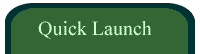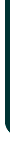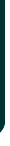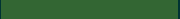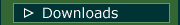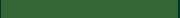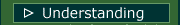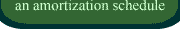VIDEOSamortizationdotcom Mortgage Calculator for iPhone

Introduction to Canadian and American Mortgages

Seminar on prepaying principal (Part A)

Seminar on prepaying principal (Part B)

Global TV Interview regarding 40 Year MortgagesLook for this logo on the Apple Store!

 < Go Back